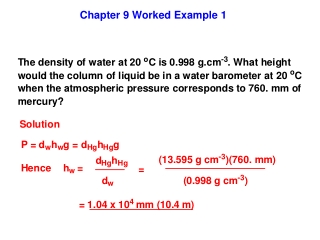DownloadDownload PresentationChapter 9 Worked Example 1

# Chapter 9 Worked Example 1

Download Presentation## Chapter 9 Worked Example 1

- - - - - - - - - - - - - - - - - - - - - - - - - - - E N D - - - - - - - - - - - - - - - - - - - - - - - - - - -
##### Presentation Transcript

1. Chapter 9 Worked Example 1

2. Chapter 9 Worked Example 2 A student attaches a glass bulb containing neon gas to an open-tube manometer and calculates the pressure of the gas to be 0.890 atm. • If the atmospheric pressure is 762 Torr, what height difference • between the two sides of the mercury in the manometer did the • student find? (b) Which side is higher, the side of the manometer attached to the bulb or the side open to the atmosphere? (c) If the student mistakenly switches the numbers for the sides of the manometer when recording the data in the laboratory notebook, what would be the reported pressure in the gas bulb?

3. Solution

4. Chapter 9 Worked Example 3

5. Chapter 9 Worked Example 4

6. Chapter 9 Worked Example 5

7. Chapter 9 Worked Example 6 A sample of methane gas, CH4, was slowly heated at a constant pressure of 0.90 bar. The volume of the gas was measured at a series of different temperatures and a plot of volume vs. temperature was constructed. The slope of the line was 2.88×10-4 L K-1. What was the mass of the sample of methane? Solution

8. Chapter 9 Worked Example 7

9. Chapter 9 Worked Example 8